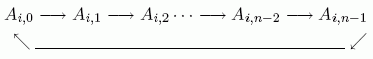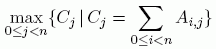# Matrix

Time Limit: Java: 2000 ms / Others: 2000 ms

Memory Limit: Java: 65536 KB / Others: 65536 KB

## Description

Given an n*n matrix A, whose entries Ai,j are integer numbers ( 0 <= i SHIFT at row i ( 0 <= iYou can do the SHIFT operation at arbitrary row, and as many times as you like. Your task is to minimize## Input

The input consists of several test cases. The first line of each test case contains an integer n. Each of the following n lines contains n integers, indicating the matrix A. The input is terminated by a single line with an integer -1. You may assume that 1 <= n <= 7 and |Ai,j| 4.

## Output

For each test case, print a line containing the minimum value of the maximum of column sums.

## Sample Input

2
4 6
3 7
3
1 2 3
4 5 6
7 8 9
-1

## Sample Output

11
15

None

## Source

Asia 2004, Shanghai (Mainland China), Prelimi